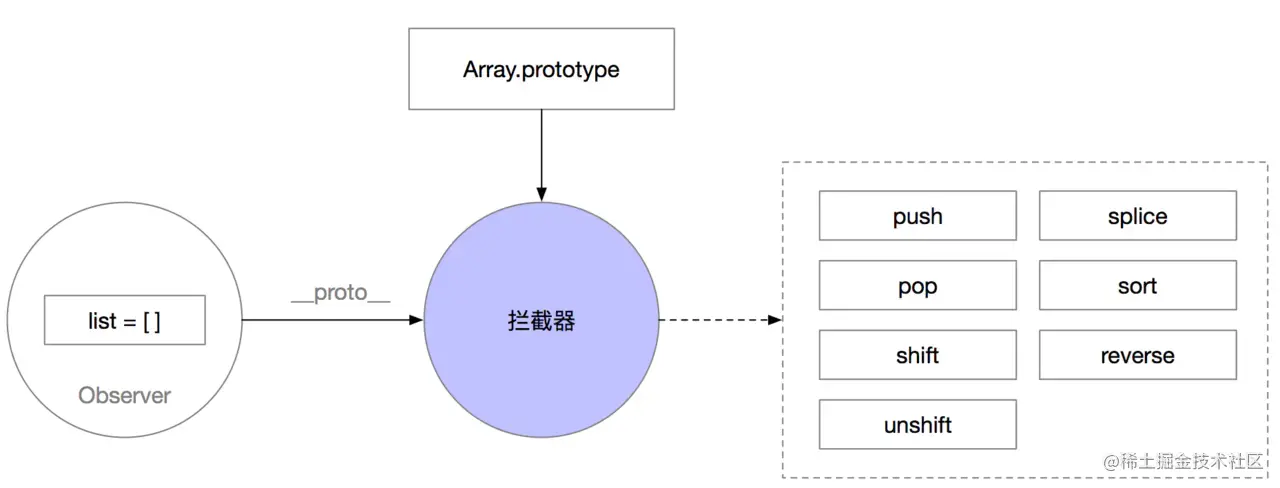# 为 react 添加双向绑定 hook

• 生态很繁荣，但是不知道那种方案是最佳
• 开发效率很繁琐（这一点待商榷）

``````// ...
const list = myContent.list[props.index]!;
list.title = inp;
list.show = false;
myContent.setlist([...myContent.list]);

``````import { useModel } from './utils';
const app = () => {
// ... 省略其他代码
const [arr] = useModel([]);
arr.push(1);
};

## Object.defineProperty

• 不支持拦截`length`属性，这点很关键，会导致我们直接修改`arr.length = 0`无效，原因是内部引擎的规定不允许监听

• 不支持方法监听，例如使用 push 等

• 约定不能直接修改`length`，例如：`arr = {}`
• 使用变异方法`push、pop、shift、unshift、splice、sort、reverse`来完成对数组的删除和其他修改；
• 允许修改存在的数组下标，可以直接修改 arr这样的数据；

## 实现思路

``````const arr = [];
const myProto = Object.create(Array.prototype);
const arrFn = arr.push;
myProto.push = (...rest) => {
consoole.log(1);
arrFn.push.apply(arr, rest);
};
Object.setPrototypeOf(arr, myProto);
// 1
arr.push(1);## 具体实现

``````import { useState } from 'react';
const isObject = (obj) => {
return (typeof obj === 'object' && obj) || typeof obj === 'function';
};
// 直接改写成一个通用遍历，这里进行类型判断，后续的useModel则不需要进行判断了
const each = (obj, change) => {
if (!isObject(obj)) {
return;
}
const isArr = Array.isArray(obj);
const keys = isArr ? obj : Object.keys(obj);
for (let i = 0, len = keys.length; i < len; i++) {
const key = isArr ? i : keys[i];
const value = obj[key];
change(key, value);
}
};
export const useModel = (obj) => {
const [model, setModel] = useState(obj);
// 更新的时候直接更新顶层对象即可，因为这是hook写法不存在class的局部替换
const setRootValue = () => {
if (Array.isArray(model)) {
setModel([...model]);
return;
}
setModel(Object.assign({...model});
};
// 定义对象的key
const defineProperty = (key, value, o) => {
Object.defineProperty(o, key, {
enumerable: true,
get() {
return value;
},
set(v) {
if (v === value || (Number.isNaN(v) && Number.isNaN(value))) {
return;
}
value = v;
setRootValue();
},
});
};
const definePropertyArray = (all) => {
const myProto = Object.create(Array.prototype);
each(['push', 'pop', 'shift', 'unshift', 'splice', 'sort', 'reverse'], (_, value) => {
const fn = all[value];
myProto[value] = (...rest) => {
fn.apply(all, rest);
setRootValue();
};
});
Object.setPrototypeOf(all, myProto);
};
const observer = (all) => {
each(all, (key, value) => {
defineProperty(key, value, all);
observer(value);
});
if (Array.isArray(all)) {
definePropertyArray(all);
}
};
observer(model);
return [model, setRootValue];
};